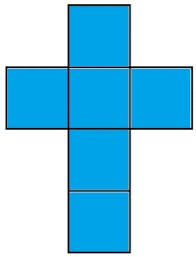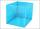# The cube

The cube has a surface area of 216 dm2. Calculate:
a) the content of one wall,
b) edge length,
c) cube volume.

S1 =  36 dm2
a =  6 dm
V =  216 dm3

### Step-by-step explanation:Did you find an error or inaccuracy? Feel free to write us. Thank you!Tips to related online calculators
Tip: Our volume units converter will help you with the conversion of volume units.

## Related math problems and questions:

• TerezaThe cube has an area of base 256 mm2. Calculate the edge length, volume, and area of its surface.
• The cubeThe cube has a surface area of 486 m ^ 2. Calculate its volume.
• Cube surface areaThe surface of the cube was originally 216 centimeters square. The surface of the cube has shrunk from 216 to 54 centimeters sq. Calculate how much percent the edge of the cube has decreased.
• Cube 6Volume of the cube is 216 cm3, calculate its surface area.
• Cube surface and volumeFind the surface of the cube with a volume of 27 dm3.
• Edge of prismThe regular quadrilateral prism has a surface of 250 dm2, its shell has a content of 200 dm2. Calculate its leading edge.
• Cube wallThe surface of the first cube wall is 64 m2. The second cube area is 40% of the surface of the first cube. Determine the length of the edge of the second cube (x).
• Cube surfce2volumeCalculate the volume of the cube if its surface is 150 cm2.
• Area to volumeIf the surface area of a cube is 486, find its volume.
• Cube V2SThe volume of the cube is 27 dm cubic. Calculate the surface of the cube.
• The cubeThe cube has a surface of 600 cm2. What is its volume?
• Volume and areaWhat is the volume of a cube which has an area of 361 cm2?
• Cube 5The surface of the cube is 15.36 dm2. How will change the surface area of this cube if the length of the edges is reduced by 2 cm?
• Length of the edgeFind the length of the edge of a cube with a cm2 surface and a volume in cm3 expressed by the same number.
• Cube 8The surface of the cube is 0.54 m2. Calculate the length of the cube edge.
• Cube containersReplace the two cube-shaped containers with 0.8 dm and 0.6 dm edges with a single cube-shaped one so that it has the same volume as the two original ones together. What is the length of the edge of this cube?
• Third dimensionCalculate the third dimension of the cuboid: a) V = 224 m3, a = 7 m, b = 4 m b) V = 216 dm3, a = 9 dm, c = 4 dm